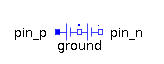Voltage Sensor - MapleSim Help

Quasistationary Multiphase Voltage Sensor

Voltage sensorDescription The Quasistationary Multiphase Voltage Sensor (or Voltage Sensor) component contains $m$ quasistationary single-phase voltage sensor models, each connected between corresponding phases of ${\mathrm{plug}}_{p}$ and ${\mathrm{plug}}_{n}$, with the outputs connected to the corresponding signal of an output vector.Connections

 Name Description Modelica ID ${\mathrm{plug}}_{p}$ Positive quasistationary multiphase plug plug_p ${\mathrm{plug}}_{n}$ Negative quasistationary multiphase plug plug_n $y$ Real vector output; voltage in $V$ yParameters

 Name Default Units Description Modelica ID $m$ $3$ Number of phases mModelica Standard Library The component described in this topic is from the Modelica Standard Library. To view the original documentation, which includes author and copyright information, click here.